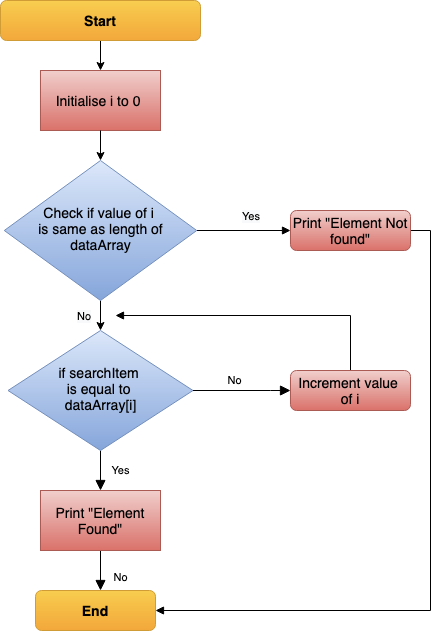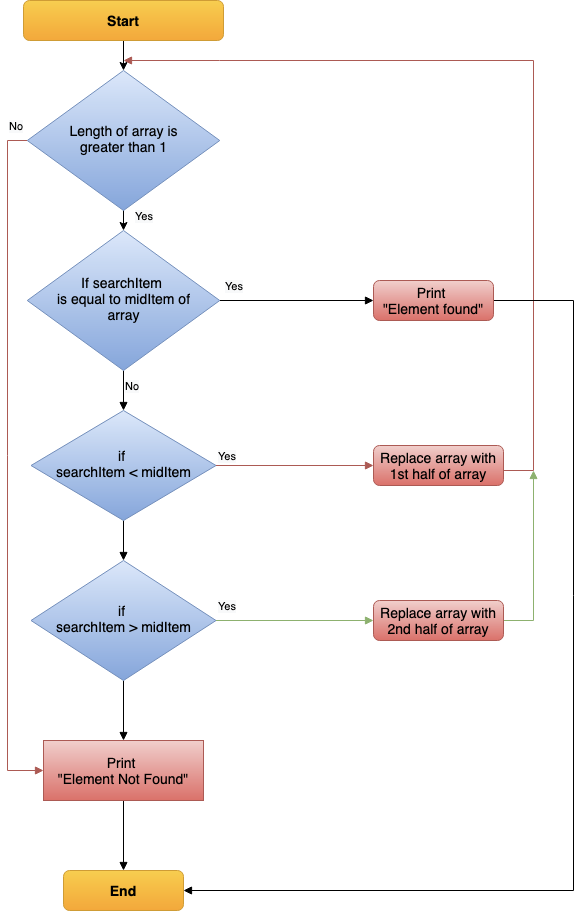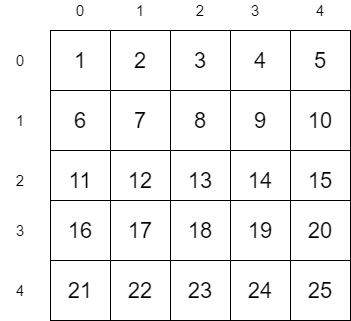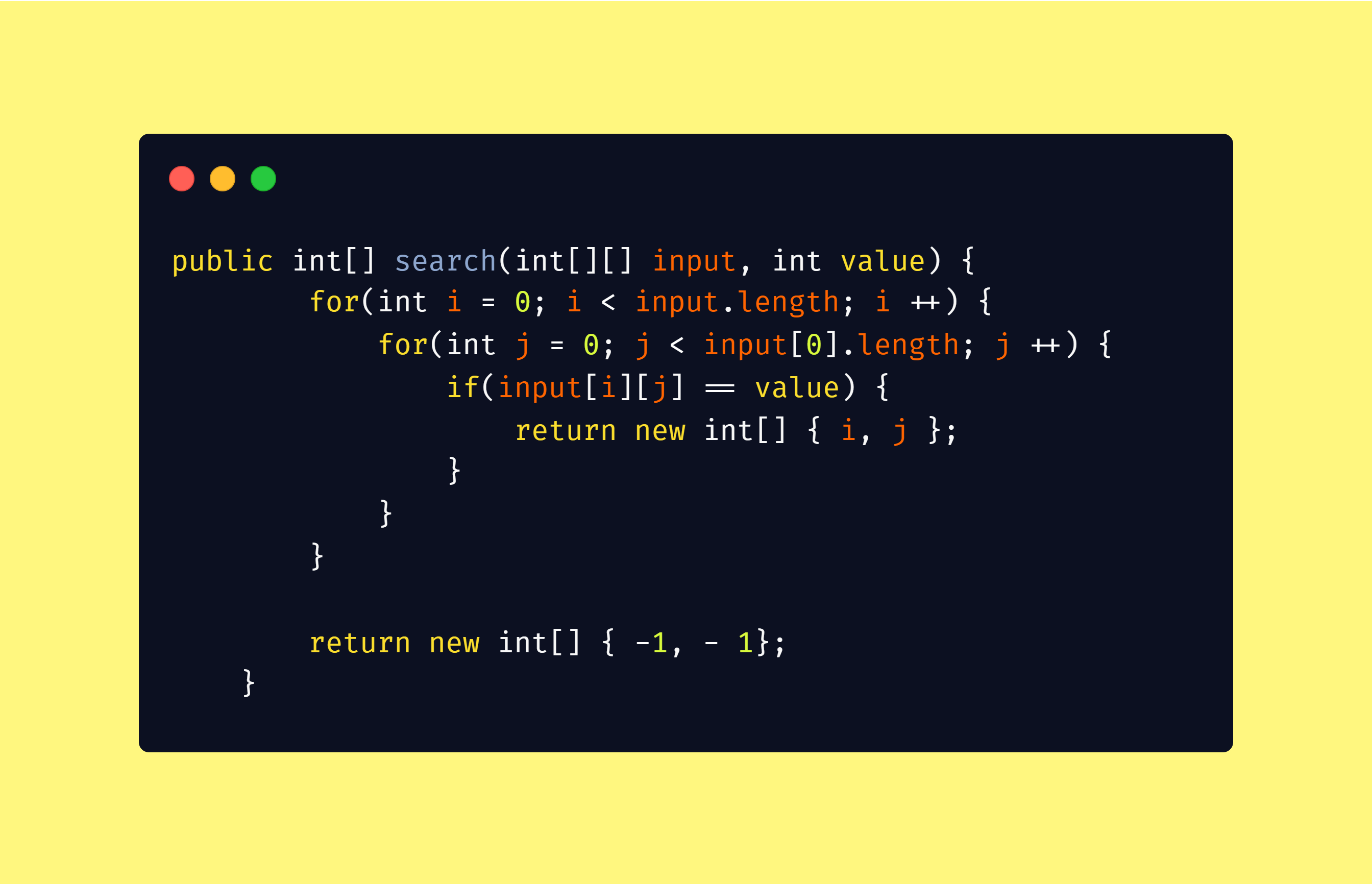1619231827

# Search Algorithm: Binary Search

Search Algorithm: Binary Search

#data-science #developer

## Buddha Community1625122494

## Binary Search in Python (Recursive and Iterative)

Searching for an element’s presence in a list is usually done using linear search and binary search. Linear search is time-consuming and memory expensive but is the simplest way to search for an element. On the other hand, Binary search is effective mainly due to the reduction of list dimension with each recursive function call or iteration. A practical implementation of binary search is autocompletion.

#### Python Binary Search Algorithm:

The objective of this project is to create a simple python program to implement binary search. It can be implemented in two ways: recursive (function calls) and iterative.

#### Project Prerequisites:

The project uses loops and functions to implement the search function. Hence good knowledge of python loops and function calls is sufficient to understand the code flow.

#python tutorials #binary search python #binary search python program #iterative binary search python #recursive binary search python1603767600

## Search Algorithms

Today, let us touch base on some fundamental concepts like search algorithms.

In simple terms, **searching **is a process of looking up a particular data record in the database or in the collection of items. A search typically answers as true or false whether the particular data in which we are referring is found or not and perform the next action accordingly.

Commonly used algorithms for search are:

• Linear search
• Binary search
• Interpolation search

Let us understand them in detail with some example

## Linear Search Algorithm

Linear Search Algorithm is the simplest and easiest form of the search algorithm. In this algorithm, a sequential search is made over all the items one by one to search for the targeted item. Each item is checked in sequence until the match is found. If the match is found, the searched item is returned otherwise the search continues till the end.

To make it easy to understand, let us see understand linear search using a flow diagramLinear Search — Data Flow

### Points to note:

• Does not need sorted array list
• Performs equality comparisons
• The time complexity is O(n)
• Time taken to search elements keeps increasing as the number of elements is increased.

## Binary Search Algorithm

In _Binary search algorithm, _begins with an interval covering the whole array and diving it in half. If the value of the search key is less than the item in the middle of the interval, narrow the interval to the lower half. Otherwise narrow it to the upper half. Repeatedly check until the value is found or the interval is empty.

To make it easy to understand, let us see understand binary search using flow diagram and example as below.Binary Search — Data Flow

### Points to note:

• The array needs to be sorted
• Performs ordering comparisons
• Time complexity to O(log n).
• Search is done to either half of the given list, thus cut down your search to half time

#sorting-algorithms #algorithms #data-structures #search-and-sort #searching-algorithm1593399960

## Leveraging The Binary Search Algorithm

Last week at work I was investigating Google’s Firebase products, in particular, the Cloud Firestore database. So, I decided to take a deep dive into the documentation pages. I learned a lot about integrating the database to an application, its data model, best practices to structure our data, and how to create queries using the available SDKs. As I was reading the…

#software-engineering #google #nosql #binary-search #algorithms #algorithms1596737760

# Statement

We have to search for a value **x **in a sorted matrix M. If **exists, then return its coordinates **(i, j), else return (-1, -1).Let us consider the above matrix as an example. In this example, we are going to search for the value 12. Since 12 is present in the matrix, the algorithm should return its coordinates (2, 1)

# Simple Approach

A simple approach is to traverse all the values in the matrix and check if it is equal to 12.The worst case time complexity of the above algorithm will be

_O(n x m) = O(n²) __when _n = m

# Efficient Approach

The above algorithm behaves worse for large values of n and m. Let us look into the efficient algorithm now.

## Algorithm

``````1\. Start from Top Right position (0, m - 1) in the matrix M
2\. If the value is equal to x return (0, m - 1)
3\. Move one row down if the current value is less than x
4\. Move one column left if the current value is greater than x
``````

Let us apply this algorithm into our matrix M. We are going to search for the value 12 in M

#data-structures #search-sorted-matrix #2d-binary-search #algorithms #matrix-search #algorithms1597045745

## Searching Algorithms in Java

Searching Algorithms are designed to check for an element or retrieve an element from any data structure where it is stored. Based on the type of search operation, these algorithms are generally classified into two categories:

1. Sequential Search: In this, the list or array is traversed sequentially and every element is checked. For Example: Linear Search.
2. Interval Search: These algorithms are specifically designed for searching in sorted data-structures. These type of searching algorithms are much more efficient than Linear Search as they repeatedly target the center of the search structure and divide the search space in half. For Example: Binary Search.

Linear Search: The idea is to traverse the given array arr[] and find the index at which the element is present. Below are the steps:

• Let the element to be search be x.
• Start from the leftmost element of arr[] and one by one compare x with each element of arr[].
• If x matches with an element then return that index.
• If x doesn’t match with any of elements then return -1.

#java #searching #algorithms-searching #binary search